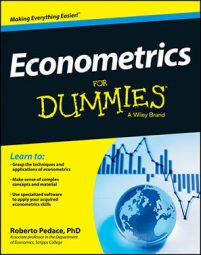##### Econometrics For DummiesIf the classical linear regression model (CLRM) doesn't work for your data because one of its assumptions doesn't hold, then you have to address the problem before you can finalize your analysis. Fortunately, one of the primary contributions of econometrics is the development of techniques to address such problems or other complications with the data that make standard model estimation difficult or unreliable.

The following table lists the names of the most common estimation issues, a brief definition of each one, their consequences, typical tools used to detect them, and commonly accepted methods for resolving each problem.

Problem Definition Consequences Detection Solution
High multicollinearity Two or more independent variables in a regression model exhibit a close linear relationship. Large standard errors and insignificant t-statistics
Coefficient estimates sensitive to minor changes in model specification
Nonsensical coefficient signs and magnitudes
Pairwise correlation coefficients
Variance inflation factor (VIF)
2. Re-specify the model.
3. Drop redundant variables.
Heteroskedasticity The variance of the error term changes in response to a change in the value of the independent variables. Inefficient coefficient estimates
Biased standard errors
Unreliable hypothesis tests
Park test
Goldfeld-Quandt test
Breusch-Pagan test
White test
1. Weighted least squares (WLS)
2. Robust standard errors
Autocorrelation An identifiable relationship (positive or negative) exists between the values of the error in one period and the values of the error in another period. Inefficient coefficient estimates
Biased standard errors
Unreliable hypothesis tests
Geary or runs test
Durbin-Watson test
Breusch-Godfrey test
1. Cochrane-Orcutt transformation
2. Prais-Winsten transformation
3. Newey-West robust standard errors